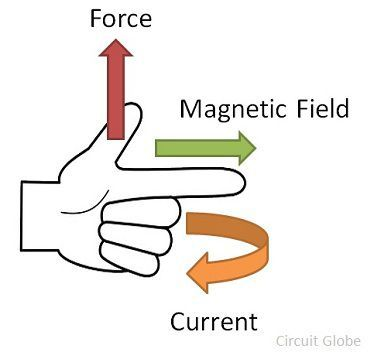# Working Principle of a DC Motor

The DC motor is the device which converts the direct current into the mechanical work. It works on the principle of Lorentz Law, which states that the current-carrying conductor placed in a magnetic and electric field experience a force”.  The experienced force is called the Lorentz force. The Flemming left-hand rule gives the direction of the force.

### Fleming Left Hand Rule

If the thumb, middle finger and the index finger of the left hand are displaced from each other by an angle of 90°, the middle finger represents the direction of the magnetic field. The index finger represents the direction of the current, and the thumb shows the direction of forces acting on the conductor.The formula calculates the magnitude of the force,Before understanding the working of DC motor, first, we have to know about its construction. The armature and stator are the two main parts of the DC motor. The armature is the rotating part, and the stator is their stationary part. The armature coil is connected to the DC supply.

The armature coil consists the commutators and brushes. The commutators convert the AC induced in the armature into DC and the brushes transfer the current from rotating part of the motor to the stationary external load. The armature is placed between the north and south pole of the permanent or electromagnet.

For simplicity, consider that the armature has only one coil which is placed between the magnetic field shown below in the figure A. When the DC supply is given to the armature coil the current starts flowing through it. This current develops its own field around the coil.

Figure B shows the field induces around the coil:By the interaction of the fields (produced by the coil and the magnet), the resultant field develops across the conductor. The resultant field tends to regain its original position, i.e. in the axis of the main field. The field exerts the force at the ends of the conductor, and thus the coil starts rotating.Let the field produced by the main field be Fm, and this field rotates in the clockwise direction. When the current flows in the coil, they produce their own magnetic field say, Fr. The field Fr tries to come in the direction of the main field. Thereby, the torque act on the armature coil.The actual DC motor consists of a large number of armature coils. The speed of the motor is directly proportional to the number of coils used in the motor. These coils are kept under the impact of the magnetic field.

The one end of the conductors is kept under the influence of the north pole, and the other end is kept under the influence of the south pole. The current enters into the armature coil through the north pole and move outwards through the south pole.

When the coil moves from one brush to another, at the same time the polarity of the coil also changes. Thus, the direction of the force or torque acting on the coil remains the same.

The torque induces in the coil become zero when the armature coil is perpendicular to the main field. The zero torque means the motor stops rotating. For solving this problem, the number of armature coil is used in the rotor. So, if one of their coils is perpendicular to the field, then the other coils induce the torque. And the rotor moves continuously.Also, for obtaining the continuous torque, the arrangement is kept in such a way that whenever the coils cut the magnetic neutral axis of the magnet the direction of current in the coils become reversed. This can be done with the help of the commutator.

### 12 thoughts on “Working Principle of a DC Motor”

1. Danushka T Rathnayake

This is very useful and clear introduction. I wish you the best to continue this work…